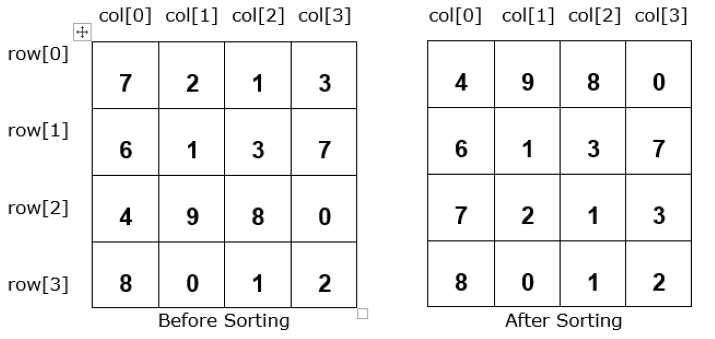# Sorting a 2D Array according to values in any given column in Java

Array is a linear data structure that is used to store a group of elements with similar datatypes. It stores data in a sequential manner. Once we create an array we can’t change its size i.e. it is of fixed length.

Suppose we have a 2D array of order M x M where M is the number of rows and columns. We have to sort the specified column of the given array. In this article, we will try to find the solution to the given problem.

## Sorting 2D Array according to column value

Sorting means rearranging the elements of a given list or array in ascending or descending order. Let’s understand what is sorting with the following visual representation −

### Example 1

When we sort the 1st column of this 2D array −### Syntax for 2D Array

// declaration with size
Data_Type nameOfarray[][] = new Data_Type[sizeofrow][sizeofcolumn];
Or,
// declaration and initialization
Data_Type nameOfarray[][] = { {values separated by comma} };


We can use any of the above syntax in our program.

At the place of Data_Type, we can give primitive data types like int and double. Row and Column are the required size of array.

Before jumping into the program let’s discuss one more thing.

### Comparator Interface

Java provides an inbuilt method named sort() that can sort arrays and collections in natural ordering. The Comparator is a generic interface that can be used when we need to sort elements in our custom ways, basically, we can control the order of sorting. This interface defines a method ‘compare()’ that takes two parameters and compares those. It returns 0 when both parameters are equal, a positive value if first value is greater than second otherwise a negative value.

### Syntax

Comparator<typeOfelement> nameOfcollection = new Comaprator<typeOfelement>() {
};


### Algorithm

• Step 1 − Define a method ‘araySort()’ along with two parameters inside class ‘Srt’. Inside this method, create an object of Comparator interface ‘comp’. Now, define compare method that will take two rows of array together as parameter.

• Step 2 − Moving further we will take an if-else block that will compare the specified column values and return 1 if element of first column is greater than second column otherwise return -1.

• Step 3 − Now, use ‘Arrays.sort()’ method to sort the array.

• Step 4 − Take two for loops to print the new sorted array.

• Step 5 − At the end, inside the main() method, we will declare and initialize an array. Moving ahead create an object of class ‘Srt’ to call the method ‘araySort()’ with ‘aray’ and column index as arguments.

### Example

import java.util.*;
class Srt {
void araySort(int aray[][], int cl) {
Comparator<int[]> comp = new Comparator<int[]>() {
public int compare(int[] val1, int[] val2) {
if(val1[cl-1] > val2[cl-1]) {
return 1;
} else {
return -1;
}
}
};
Arrays.sort(aray, comp);
System.out.println("The sorted array: ");
for(int i = 0; i< aray.length; i++) {
for (int j = 0; j < aray[i].length; j++) {
System.out.print(aray[i][j] + " ");
}
System.out.println();
}
}
}
public class Sorting {
public static void main(String[] args) {
int aray[][] = { { 7, 2, 1, 3 }, { 6, 1, 3, 7 }, { 4, 9, 8, 0 }, { 8, 0, 1, 2 } };
System.out.println("The given array we have: ");
// for each loop to print original 2D array
for (int[] array : aray) {
for (int print : array) {
System.out.print(print + " ");
}
System.out.println();
}
Srt obj = new Srt();
// calling method using object
obj.araySort(aray, 1);
obj.araySort(aray, 3);
}
}


### Output

The given array we have:
7 2 1 3
6 1 3 7
4 9 8 0
8 0 1 2
The sorted array:
4 9 8 0
6 1 3 7
7 2 1 3
8 0 1 2
The sorted array:
8 0 1 2
7 2 1 3
6 1 3 7
4 9 8 0


## Conclusion

The 2D array is an array with rows and columns. In this article, we have created a Java program to sort 2D array according to the values of specified column. We understood how we can use the inbuilt method ‘compare()’ of Comparator Interface to sort an array or collection.

Updated on: 12-May-2023

350 Views